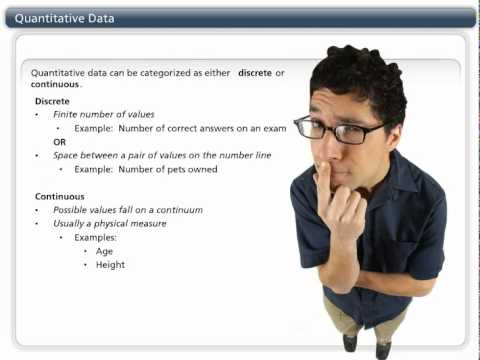### Main Difference

The statistics are the practice or science of collecting and analyzing numerical data in large quantities. It is especially assigned for the purpose of inferring proportions in a whole from those in a representative sample. The statistic is quite handy in the arrangement and collection of the data. Mainly the numerical or the data in the number value is evaluated in the statistics. There are several types of the data that are gathered and analyzed. The notable techniques of data analyzation are primary data, secondary data, qualitative data and the quantitative data. The qualitative data is the type of data which in properties and attributes do the data collection. The representation of qualitative data is done with the language or words, and not with the numbers or the numerical values. Conversely, the quantitative data is the type of the data in which the data classification is done by the quantity, amount or the range. The quantitative data is expressed using the number, measurement units or the numerical values.

### Comparison Chart

 Qualitative Data Quantitative Data Definition The qualitative data is the type of data that can be observed but can’t be measured. The quantitative data is the one which is represented using the numbers, numerical values, and the measurement units. Properties Texture, taste, feel, smell is few of the observable properties that are used in this qualitative data interpretation. The data is classified into different groups by the quantity, amount, or range in qualitative data. Nature There is non-statistical analysis for the qualitative data. Statistical analysis is used for the quantitative data. Representation In qualitative data words are used for expression. In quantitative data, the numbers and measuring units are used for expression.

### What is Qualitative Data?

The qualitative data is the type of data that can be observed but can’t be measured. The object classification in this type of data is done with regards to the attributes and properties. It is the approximate data analysis, which can’t be computed or that accurate. The one analyzing this type of data needs to have the prior knowledge about the types of objects and their characteristics. If any of the laypeople tries to analyze it, the things can get worse as the qualitative data is descriptive in nature, and when it comes to analyzing it requires some expert approach.During the data analyzation process, the objects are placed into different categories after they are distinguished by the physical attributes and the properties of the object. The data interpretation is purely based on the observation and the properties, which can be observed but can’t express using the numbers. In a more compact way, we can say that is the type of data interpretation in which language and words are used for the arrangement and analyzing the data. Texture, taste, feel, smell is few of the observable properties that are used in this type of data interpretation. Other than observations, the qualitative data is based on the interviews or evaluations. The qualitative data is also called the categorical data as the information is classified by category and not by the numbers.

### What is Quantitative Data?

The quantitative data is the one which is represented using the numbers, numerical values, and the measurement units. The data is classified into different groups by the quantity, amount, or range. In other words, we can say that it is the number game on which the different arithmetic operations can also apply, and the validity can also be checked for it. The quantitative data is the method in which data is numerically counted or expressed. Table charts, graphs, histograms are even used here for the purpose of expressions. With using the above mentioned, the data evaluation for one gets quite easy as it covers the all in the very concise way. The measuring of length, volume, area, and temperature are few of the prominent examples of this type of data analyzation. The numbers are required in this case along with the measuring units. Experiments, surveys, and observations are conducted in this type of data evaluation.

### Qualitative Data vs. Quantitative Data

• The qualitative data is the type of data that can be observed but can’t be measured. On the other hand, The quantitative data is the one which is represented using the numbers, numerical values, and the measurement units.
• Texture, taste, feel, smell is few of the observable properties that are used in this qualitative data interpretation. Contrary to this, The data is classified into different groups by the quantity, amount, or range in qualitative data.
• There is non-statistical analysis for the qualitative data while statistical analysis is used for the quantitative data.
• In quantitative data, the numbers and measuring units are used for expression, whereas in qualitative data words are used for expression.